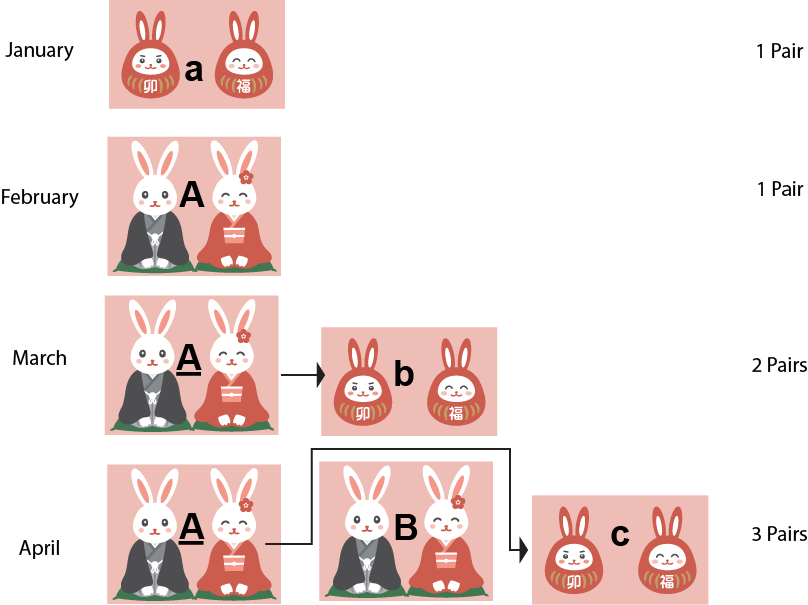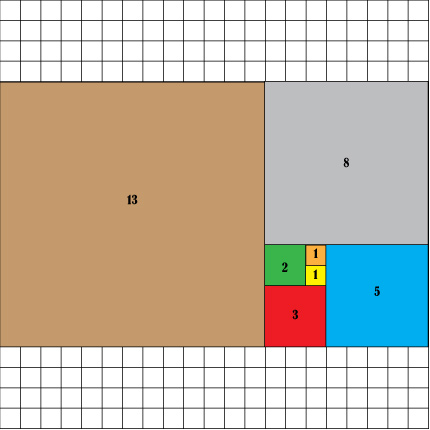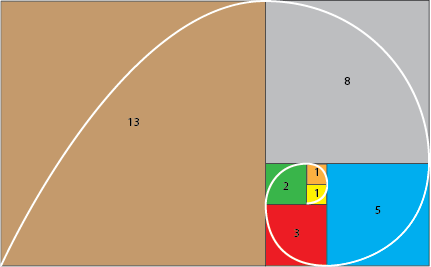# 2023: Year of the Rabbit

This is the year of the rabbit in the Chinese zodiac. This reminds us of the Fibonacci rabbits that were used to generate the Fibonacci sequence, one of the most important number patterns.Media4Math is building a series of lessons on the Fibonacci sequence to give your students a better understanding of a number of related topics. In the lessons we will cover the following topics:

 Lesson 1: The Basics of the Fibonacci Sequence In this lesson students learn about what the Fibonacci sequence is by investigating the pattern formed by rabbit generations. We provide clear graphics. We build on this lesson with the subsequent lessons.Lesson 2: Visualizing Fibonacci Sequence Having established the numerical pattern that makes up the Fibonacci sequence, we then go through a visualization of it. This lays the groundwork for building a logarithmic spiral in the next lesson.Lesson 3: Logarithmic Spirals In this lesson we look at logarithmic spirals, as well as other types of spirals. This provides an opportunity to express the polar coordinate system.